# 5 分钟教你搭建「视频动作分类」系统

• 2022-11-16
北京
• 本文字数：4354 字

阅读完需：约 14 分钟## 写在前面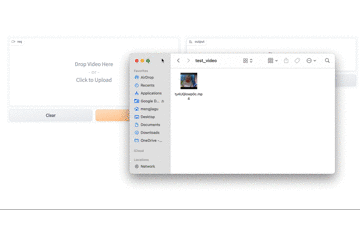「视频动作分类」系统展示图

## 准备工作

### 安装依赖包

• Towhee：用于构建模型推理流水线的框架，对于新手非常友好。

• Milvus：用于存储向量并创建索引的数据库，简单好上手。

• Pillow：图像处理常用的 Python 库。

python -m pip install -q pymilvus towhee towhee.models pillow ipython gradio

### 数据准备

curl -L https://github.com/towhee-io/examples/releases/download/data/reverse_video_search.zip -Ounzip -q -o reverse_video_search.zip

1. train：视频所在文件夹，包含 20 个类别（每个类别为一个子文件夹），每个类别包含了 10 个视频，总计有 200 个视频。

2. reverse_video_search.csv：一个 csv 文件，其中包含视频文件夹中每个视频的 id、path 和 label。接着，我们输入以下代码便可查看数据集内的详细信息：

import pandas as pddf = pd.read_csv('./reverse_video_search.csv')print(df.head(3))print(df.label.value_counts())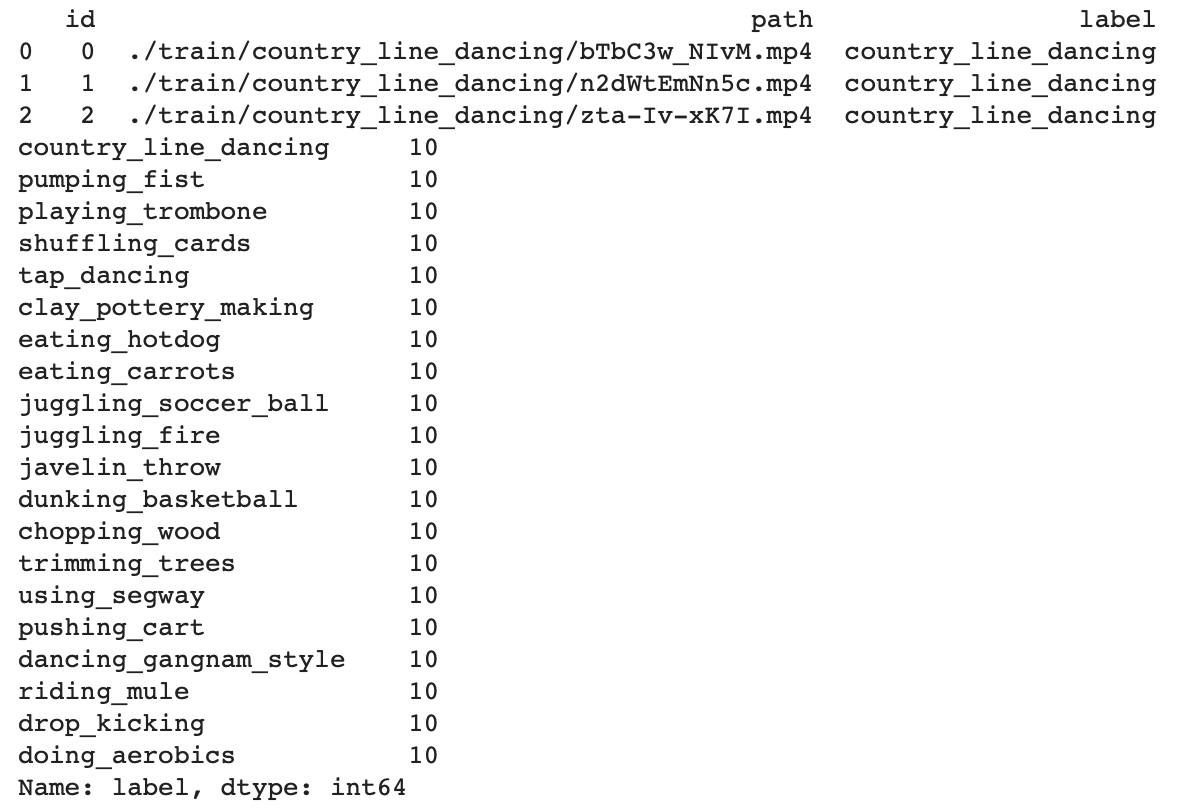def ground_truth(path):    label = df.set_index('path').at[path, 'label']    return [label.replace('_', ' ')]

## 系统搭建

### 预测类别

import towhee(    towhee.glob['path']('./train/tap_dancing/*.mp4')          .video_decode.ffmpeg['path', 'frames'](sample_type='uniform_temporal_subsample', args={'num_samples': 16})          .action_classification['frames', ('predicts', 'scores', 'features')]          .pytorchvideo(model_name='x3d_m', skip_preprocess=True, topk=5).select['path', 'predicts', 'scores']()          .show())

• towhee.read_csv()：从 CSV 文件中读取数据。

• .video_decode.ffmpeg()：一个 Towhee 的算子，能通过文件路径读取视频，并通过指定的采样方法得到一定数量的视频帧。了解更多

• .action_classification.pytorchvideo()：一个 Towhee 的算子，可以提取视频特征并预测视频所属的动作分类。了解更多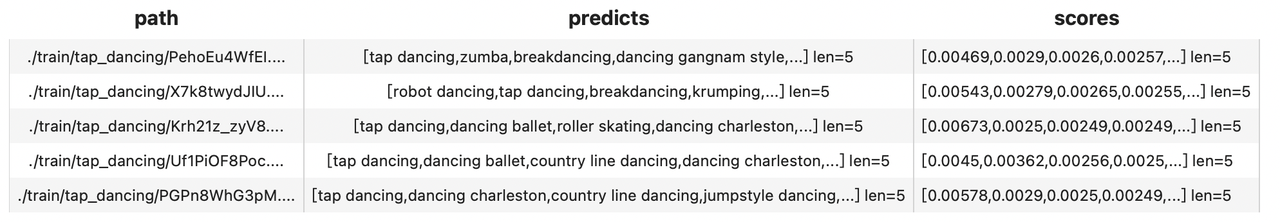### 评估和优化

import timestart = time.time()dc = (      towhee.read_csv('reverse_video_search.csv').unstream()            .video_decode.ffmpeg['path', 'frames'](sample_type='uniform_temporal_subsample', args={'num_samples': 16})            .action_classification['frames', ('predicts', 'scores', 'features')].pytorchvideo(                 model_name='x3d_m', skip_preprocess=True, topk=5))end = time.time()print(f'Total time: {end-start}')    benchmark = (      dc.runas_op['path', 'ground_truth'](func=ground_truth)        .runas_op['predicts', 'top1'](func=lambda x: x[:1])        .runas_op['predicts', 'top3'](func=lambda x: x[:3])        .with_metrics(['mean_hit_ratio'])        .evaluate['ground_truth', 'top1'](name='top1')        .evaluate['ground_truth', 'top3'](name='top3')        .evaluate['ground_truth', 'predicts'](name='top5')        .report())

Total time: 39.41930913925171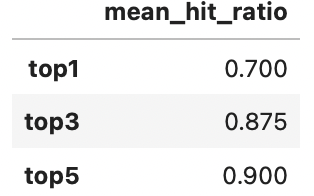import timestart = time.time()dc = (towhee.read_csv('reverse_video_search.csv').unstream()      .video_decode.ffmpeg['path', 'frames'](sample_type='uniform_temporal_subsample', args={'num_samples': 32})      .action_classification['frames', ('predicts', 'scores', 'features')].pytorchvideo(           model_name='mvit_base_32x3', skip_preprocess=True, topk=5))end = time.time()print(f'Total time: {end-start}')benchmark = (    dc.runas_op['path', 'ground_truth'](func=ground_truth)      .runas_op['predicts', 'top1'](func=lambda x: x[:1])      .runas_op['predicts', 'top3'](func=lambda x: x[:3])      .with_metrics(['mean_hit_ratio'])      .evaluate['ground_truth', 'top1'](name='top1')      .evaluate['ground_truth', 'top3'](name='top3')      .evaluate['ground_truth', 'predicts'](name='top5')      .report())

Total time: 79.16032028198242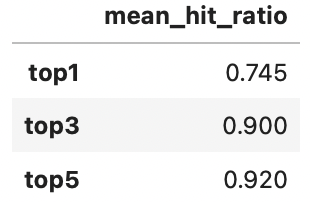start = time.time()dc =(    towhee.read_csv('reverse_video_search.csv').unstream()          .set_parallel(5)          .video_decode.ffmpeg['path', 'frames'](sample_type='uniform_temporal_subsample', args={'num_samples': 16})          .action_classification.pytorchvideo['frames', ('predicts', 'scores', 'features')](               model_name='x3d_m', skip_preprocess=True, topk=5))end = time.time()print(f'Total time: {end-start}')

### 异常处理

Towhee 支持异常处理的执行模式，允许流水线继续处理并用 Empty 值表示异常。 用户可以选择如何处理流水线末端的空值。

(    towhee.glob['path']('./exception/*')          .exception_safe()          .video_decode.ffmpeg['path', 'frames'](sample_type='uniform_temporal_subsample', args={'num_samples': 16})          .action_classification['frames', ('labels', 'scores', 'vec')].pytorchvideo(               model_name='x3d_m', skip_preprocess=True)          .drop_empty()          .select['path', 'labels']()          .show())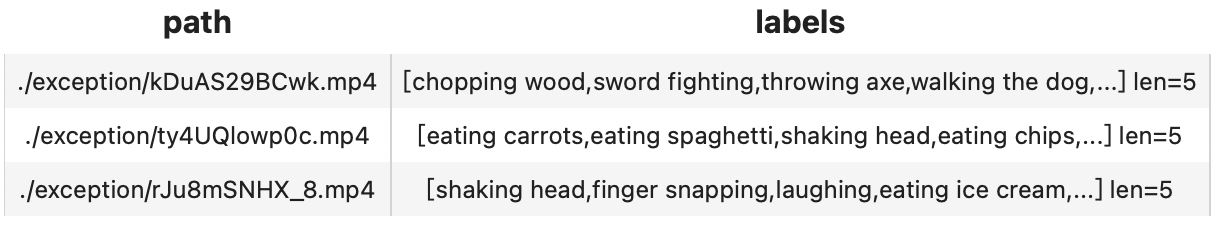Towhee 提供的 towhee.api()可以将流水线包装成一个函数，以便使用。 我们可以在 Gradio 中使用这个 action_classification_function 轻而易举地构建一个简单可交互的在线演示 demo。

import gradiotopk = 3with towhee.api() as api:    action_classification_function = (        api.video_decode.ffmpeg(            sample_type='uniform_temporal_subsample', args={'num_samples': 32})        .action_classification.pytorchvideo(model_name='mvit_base_32x3', skip_preprocess=True, topk=topk)        .runas_op(func=lambda res: {res[i]: res[i] for i in range(len(res))})        .as_function()    )            interface = gradio.Interface(action_classification_function,                                       inputs=gradio.Video(source='upload'),                                      outputs=[gradio.Label(num_top_classes=topk)]                                      )        interface.launch(inline=True, share=True)

Gradio 为我们提供了一个 Web UI，点击 URL 进行访问（或直接与 notebook 下方出现的界面进行交互）：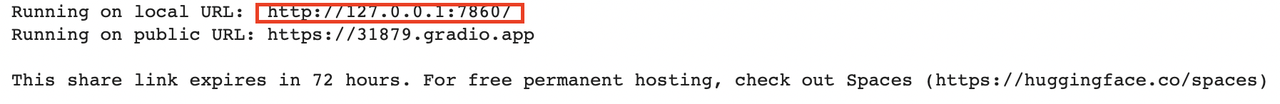## 总结### Zilliz

Reinvent data science 2021-10-09 加入

## 评论